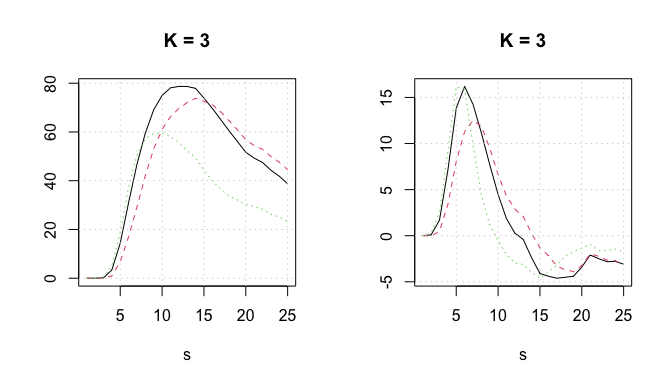# Functional PCA in R

## Overview

This is a brief introduction to the package fdapace (Gajardo et al. 2021). For a general overview on functional data analysis (FDA) see (Wang, Chiou, and Müller 2016) and key references for the PACE approach and the associated dynamics are (Yao et al. 2003; Yao, Müller, and Wang 2005; Liu and Müller 2009; Müller and Yao 2010; Li and Hsing 2010; Zhang and Wang 2016; Zhang and Wang 2018). The basic work-flow behind the PACE approach for sparse functional data is as follows (see e.g. (Yao, Müller, and Wang 2005; Liu and Müller 2009) for more information):

1. Calculate the smoothed mean $$\hat{\mu}$$ (using local linear smoothing) aggregating all the available readings together.
2. Calculate for each curve separately its own raw covariance and then aggregate all these raw covariances to generate the sample raw covariance.
3. Use the off-diagonal elements of the sample raw covariance to estimate the smooth covariance.
4. Perform eigenanalysis on the smoothed covariance to obtain the estimated eigenfunctions $$\hat{\phi}$$ and eigenvalues $$\hat{\lambda}$$, then project that smoothed covariance on a positive semi-definite surface (Hall, Müller, and Yao 2008).
5. Use Conditional Expectation (PACE step) to estimate the corresponding scores $$\hat{\xi}$$. ie. $$\hat{\xi}_{ik} = \hat{E}[\hat{\xi}_{ik}|Y_i] = \hat{\lambda}_k \hat{\phi}_{ik}^T \Sigma_{Y_i}^{-1}(Y_i-\hat{\mu}_i)$$.

As a working assumption a dataset is treated as sparse if it has on average less than 20, potentially irregularly sampled, measurements per subject. A user can manually change the automatically determined dataType if that is necessary. For densely observed functional data simplified procedures are available to obtain the eigencomponents and associated functional principal components scores (see eg. (Castro, Lawton, and Sylvestre 1986) for more information). In particular in this case we:

1. Calculate the cross-sectional mean $$\hat{\mu}$$.
2. Calculate the cross-sectional covariance surface (which is guaranteed to be positive semi-definite).
3. Perform eigenanalysis on the covariance to estimate the eigenfunctions $$\hat{\phi}$$ and eigenvalues $$\hat{\lambda}$$.
4. Use numerical integration to estimate the corresponding scores $$\hat{\xi}$$. ie. $$\hat{\xi}_{ik} = \int_0^T [ y(t) - \hat{\mu}(t)] \phi_i(t) dt$$

In the case of sparse FPCA the most computational intensive part is the smoothing of the sample’s raw covariance function. For this, we employ a local weighted bilinear smoother.

A sibling MATLAB package for fdapace can be found here.

## FPCA in R using fdapace

The simplest scenario is that one has two lists yList and tList where yList is a list of vectors, each containing the observed values $$Y_{ij}$$ for the $$i$$th subject and tList is a list of vectors containing corresponding time points. In this case one uses:

FPCAobj <- FPCA(Ly=yList, Lt=tList)

The generated FPCAobj will contain all the basic information regarding the desired FPCA.

### Generating a toy dense functional dataset from scratch

  library(fdapace)

# Set the number of subjects (N) and the
# number of measurements per subjects (M)
N <- 200;
M <- 100;
set.seed(123)

# Define the continuum
s <- seq(0,10,length.out = M)

# Define the mean and 2 eigencomponents
meanFunct <- function(s) s + 10*exp(-(s-5)^2)
eigFunct1 <- function(s) +cos(2*s*pi/10) / sqrt(5)
eigFunct2 <- function(s) -sin(2*s*pi/10) / sqrt(5)

# Create FPC scores
Ksi <- matrix(rnorm(N*2), ncol=2);
Ksi <- apply(Ksi, 2, scale)
Ksi <- Ksi %*% diag(c(5,2))

# Create Y_true
yTrue <- Ksi %*% t(matrix(c(eigFunct1(s),eigFunct2(s)), ncol=2)) + t(matrix(rep(meanFunct(s),N), nrow=M))

### Running FPCA on a dense dataset

  L3 <- MakeFPCAInputs(IDs = rep(1:N, each=M), tVec=rep(s,N), t(yTrue))
FPCAdense <- FPCA(L3$Ly, L3$Lt)

# Plot the FPCA object
plot(FPCAdense)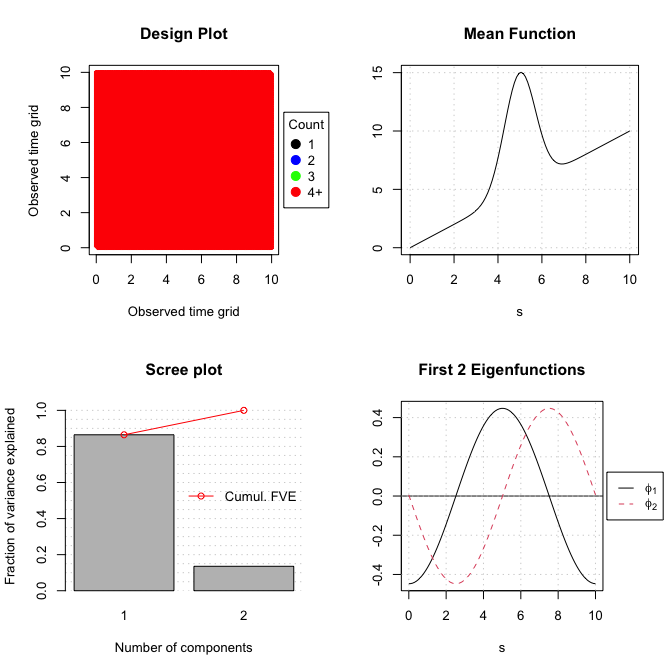# Find the standard deviation associated with each component
sqrt(FPCAdense$lambda) ##  5.050606 1.999073 ### Running FPCA on a sparse and noisy dataset  # Create sparse sample # Each subject has one to five readings (median: 3) set.seed(123) ySparse <- Sparsify(yTrue, s, sparsity = c(1:5)) # Give your sample a bit of noise ySparse$yNoisy <- lapply(ySparse$Ly, function(x) x + 0.5*rnorm(length(x))) # Do FPCA on this sparse sample # Notice that sparse FPCA will smooth the data internally (Yao et al., 2005) # Smoothing is the main computational cost behind sparse FPCA FPCAsparse <- FPCA(ySparse$yNoisy, ySparse$Lt, list(plot = TRUE))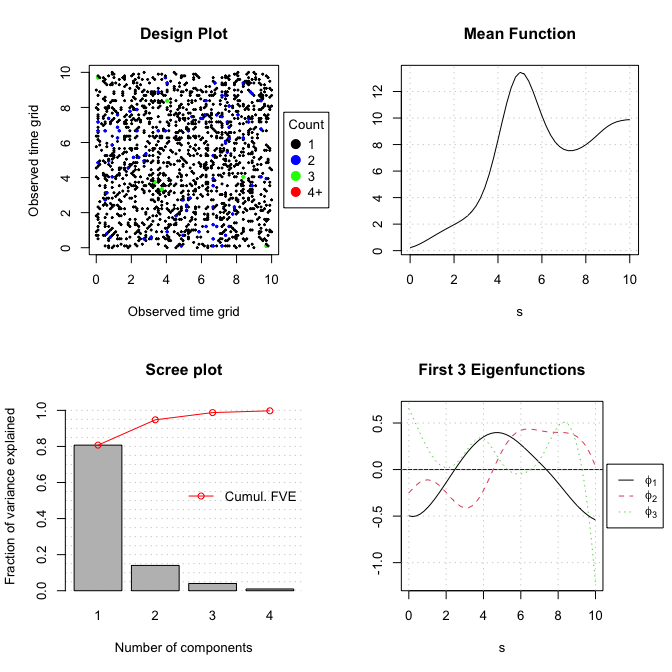## Further functionality FPCA calculates the bandwidth utilized by each smoother using generalised cross-validation or $$k$$-fold cross-validation automatically. Dense data are not smoothed by default. The argument methodMuCovEst can be switched between smooth and cross-sectional if one wants to utilize different estimation techniques when work with dense data. The bandwidth used for estimating the smoothed mean and the smoothed covariance are available under ...bwMu and bwCov respectively. Users can nevertheless provide their own bandwidth estimates:  FPCAsparseMuBW5 <- FPCA(ySparse$yNoisy, ySparse$Lt, optns= list(userBwMu = 5)) Visualising the fitted trajectories is a good way to see if the new bandwidth made any sense: par(mfrow=c(1,2)) CreatePathPlot( FPCAsparse, subset = 1:3, main = "GCV bandwidth", pch = 16) CreatePathPlot( FPCAsparseMuBW5, subset = 1:3, main = "User-defined bandwidth", pch = 16) ##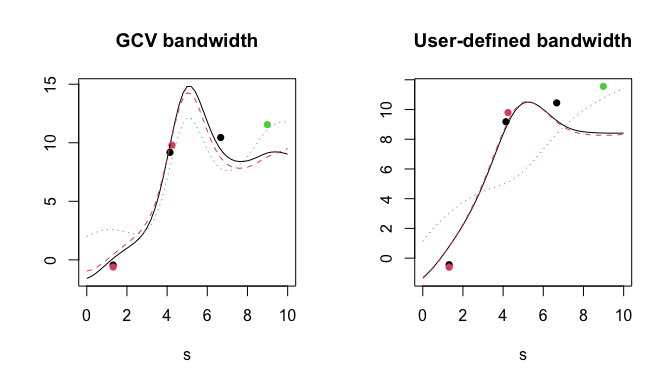FPCA uses a Gaussian kernel when smoothing sparse functional data; other kernel types (eg. Epanechnikov/epan) are also available (see ?FPCA). The kernel used for smoothing the mean and covariance surface is the same. It can be found under $optns\$kernel of the returned object. For instance, one can switch the default Gaussian kernel (gauss) for a rectangular kernel (rect) as follows:  FPCAsparseRect <- FPCA(ySparse$yNoisy, ySparse$Lt, optns = list(kernel = 'rect')) # Use rectangular kernel FPCA returns automatically the smallest number of components required to explain 99% of a sample’s variance. Using the function selectK one can determine the number of relevant components according to AIC, BIC or a different Fraction-of-Variance-Explained threshold. For example: SelectK( FPCAsparse, criterion = 'FVE', FVEthreshold = 0.95) # K = 2 ##$K
##  3
##
## $criterion ##  0.9876603 SelectK( FPCAsparse, criterion = 'AIC') # K = 2 ##$K
##  2
##
## $criterion ##  993.4442 When working with functional data (usually not very sparse) the estimation of derivatives is often of interest. Using fitted.FPCA one can directly obtain numerical derivatives by defining the appropriate order p; fdapace provides for the first two derivatives ( p =1 or 2). Because the numerically differentiated data are smoothed the user can define smoothing specific arguments (see ?fitted.FPCA for more information); the derivation is done by using the derivative of the linear fit. Similarly using the function FPCAder , one can augment an FPCA object with functional derivatives of a sample’s mean function and eigenfunctions. fittedCurvesP0 <- fitted(FPCAsparse) # equivalent: fitted(FPCAsparse, derOptns=list(p = 0)); # Get first order derivatives of fitted curves, smooth using Epanechnikov kernel fittedCurcesP1 <- fitted(FPCAsparse, derOptns=list(p = 1, kernelType = 'epan')) ## Warning in fitted.FPCA(FPCAsparse, derOptns = list(p = 1, kernelType = "epan")): Potentially you use too many components to estimate derivatives. ## Consider using SelectK() to find a more informed estimate for 'K'. ## A real-world example We use the medfly25 dataset that this available with fdapace to showcase FPCA and its related functionality. medfly25 is a dataset containing the eggs laid from 789 medflies (Mediterranean fruit flies, Ceratitis capitata) during the first 25 days of their lives. It is a subset of the dataset used by Carey at al. (1998) (Carey et al. 1998); only flies having lived at least 25 days are shown. The data are rather noisy, dense and with a characteristic flat start. For that reason in contrast with above we will use a smoothing estimating procedure despite having dense data.  # load data data(medfly25) # Turn the original data into a list of paired amplitude and timing lists Flies <- MakeFPCAInputs(medfly25$ID, medfly25$Days, medfly25$nEggs)
fpcaObjFlies <- FPCA(Flies$Ly, Flies$Lt, list(plot = TRUE, methodMuCovEst = 'smooth', userBwCov = 2))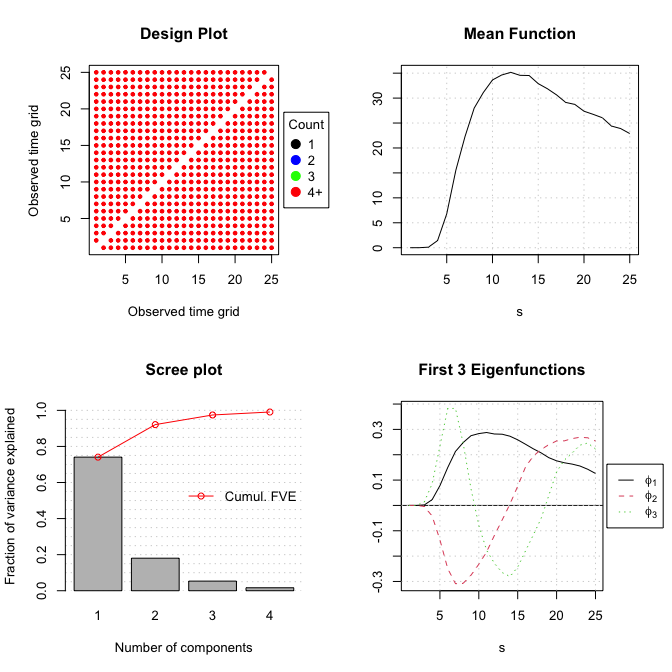Based on the scree-plot we see that the first three components appear to encapsulate most of the relevant variation. The number of eigencomponents to reach a 99.99% FVE is $$11$$ but just $$3$$ eigencomponents are enough to reach a 95.0%. We can easily inspect the following visually, using the CreatePathPlot command.

require('ks')
## Loading required package: ks
par(mfrow=c(1,2))
CreatePathPlot(fpcaObjFlies, subset = c(3,5,135), main = 'K = 11', pch = 4); grid()
CreatePathPlot(fpcaObjFlies, subset = c(3,5,135), K = 3, main = 'K = 3', pch = 4) ; grid()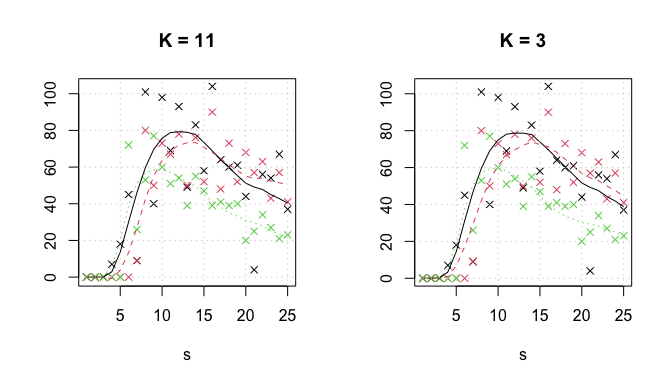One can perform outlier detection (Febrero, Galeano, and González-Manteiga 2007) as well as visualize data using a functional box-plot. To achieve these tasks one can use the functions CreateOutliersPlot and CreateFuncBoxPlot. Different ranking methodologies (KDE, bagplot Hyndman and Shang (2010) or point-wise) are available and can potentially identify different aspects of a sample. For example here it is notable that the kernel density estimator KDE variant identifies two main clusters within the main body of sample. By construction the bagplot method would use a single bag and this feature would be lost. Both functions return a (temporarily) invisible copy of a list containing the labels associated with each of sample curve0 .CreateOutliersPlot returns a (temporarily) invisible copy of a list containing the labels associated with each of sample curve.

par(mfrow=c(1,1))
CreateOutliersPlot(fpcaObjFlies, optns = list(K = 3, variant = 'KDE'))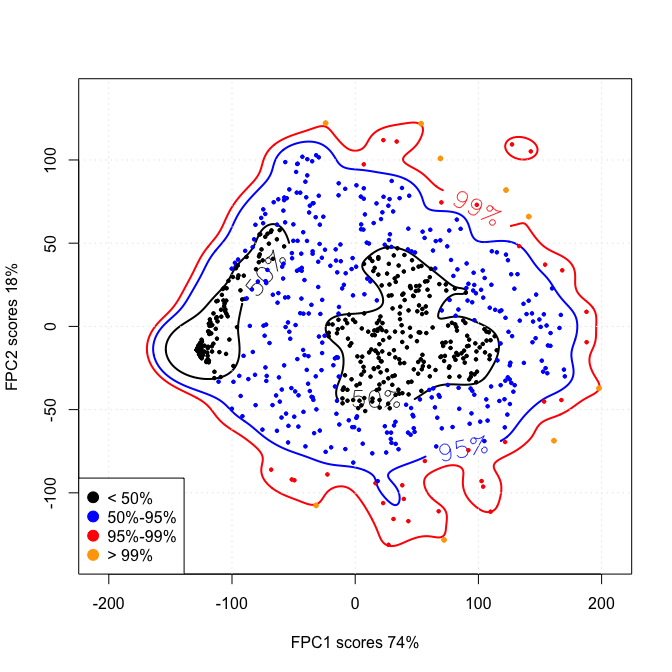CreateFuncBoxPlot(fpcaObjFlies, xlab = 'Days', ylab = '# of eggs laid', optns = list(K =3, variant='bagplot'))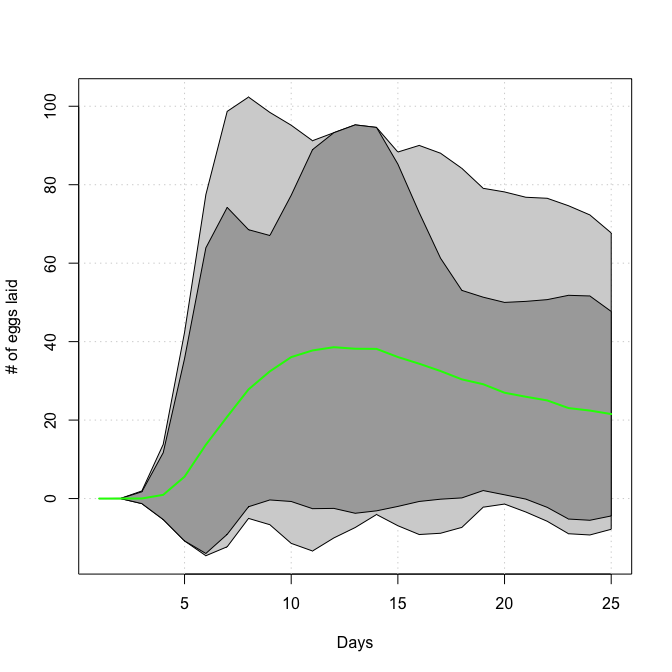Functional data lend themselves naturally to questions about their rate of change; their derivatives. As mentioned previously using fdapace one can generate estimates of the sample’s derivatives ( fitted.FPCA) or the derivatives of the principal modes of variation (FPCAder). In all cases, one defines a derOptns list of options to control the derivation parameters. Getting derivatives is obtained by using a local linear smoother as above.

par(mfrow=c(1,2))
CreatePathPlot(fpcaObjFlies, subset = c(3,5,135), K = 3, main = 'K = 3', showObs = FALSE) ; grid()
CreatePathPlot(fpcaObjFlies, subset = c(3,5,135), K = 3, main = 'K = 3', showObs = FALSE, derOptns = list(p = 1, bw = 1.01 , kernelType = 'epan') ) ; grid()